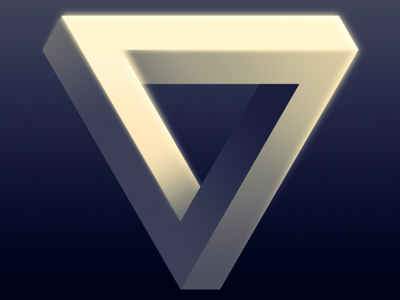We call a regular triangle an equilateral triangle.

# Shapes 2 (Difficult)

Welcome to the second of our Difficult Eleven plus maths quizzes on Shapes. If you’ve made it this far then you should breeze through these questions. They are a mixture on terms used to describe 2-D and 3-D shapes.

There are many different shapes that you need to learn. Yes, there are the obvious ones like circles, squares, triangles and cubes. But there are some more obscure ones such as trapeziums, rhomboids, parallelograms and ellipses. You need to know exactly what these shapes look like and some of their properties.

If you need more practice with shapes then fear not! Just play this quiz and all of the others on shapes until you get all the questions right first time. The more you play, the more you learn so keep at it!

Now let's see how well you can do - good luck!

1.
Which shape has the following properties?
All its interior angles are 120o
The sum of its interior angles is 720o
It can be split into six equilateral triangles
It has six vertices
A regular hexagon
A regular pentagon
A regular triangle
A hexagon is a polygon with six sides and six corners
2.
What is the name of the shape that has the following properties?
It is a polyhedron
It has two ends called “bases”
Its bases are the same size and shape
It has three or more straight edges
A pyramid
A prism
A tube
A cylinder
Cylinders are similar to prisms, but they have curved edges
3.
What is the sum of the interior angles of a triangle?
180o
360o
540o
720o
The sum of the interior angles of a quadrilateral is 360o. You can cut a quadrilateral in half to make two triangles: 360o ÷ 2 = 180o
4.
What is the name of the shape that has the following properties?
It has six faces
All its internal angles are 90o
Its height, width and depth are all equal
It has eight vertices
A pyramid
A square
A prism
A cube
A cube is, of course, a 3-D square
5.
What is another name for an oval shape?
Arc
Sector
Curve
Ellipse
An ellipse is a curved shape, similar to a squashed circle
6.
What do we call a regular triangle?
A right-angle triangle
An equilateral triangle
An isosceles triangle
A scalene triangle
Equilateral triangles have sides of equal length and interior angles of 60o
7.
Which of the following shapes not a quadrilateral?
Square
Cube
Trapezium
Kite
Quadrilaterals are 2-D shapes with four sides. There are plenty of different quadrilaterals: kites parallelograms, rhomboids, rectangles, oblongs, squares, and trapeziums for example
8.
What is the name of the shape that has the following properties?
It has two pairs of opposite sides equal in length
It has two pairs of opposite angles equal in size but not 90o
Kite
Trapezium
Rectangle
Parallelogram
The word "parallelogram" comes from the Greek for “bounded by parallel lines”.
Rectangles are parallelograms in which the internal angles are equal to 90o
9.
What is the name of the shape that has the following properties?
All points on its surface are the same distance from its centre
The distance from its centre to its surface is called its radius
The formula for its surface area is 4πr2
It is perfectly symmetrical around its centre
A circle
An ellipse
A sphere
A cytlinder
The formula for the area of a circle is πr2
10.
What is the name given to a five-sided shape whose sides are all the same length and whose interior angles are all the same size?
A regular heptagon
A regular hexagon
A regular pentagon